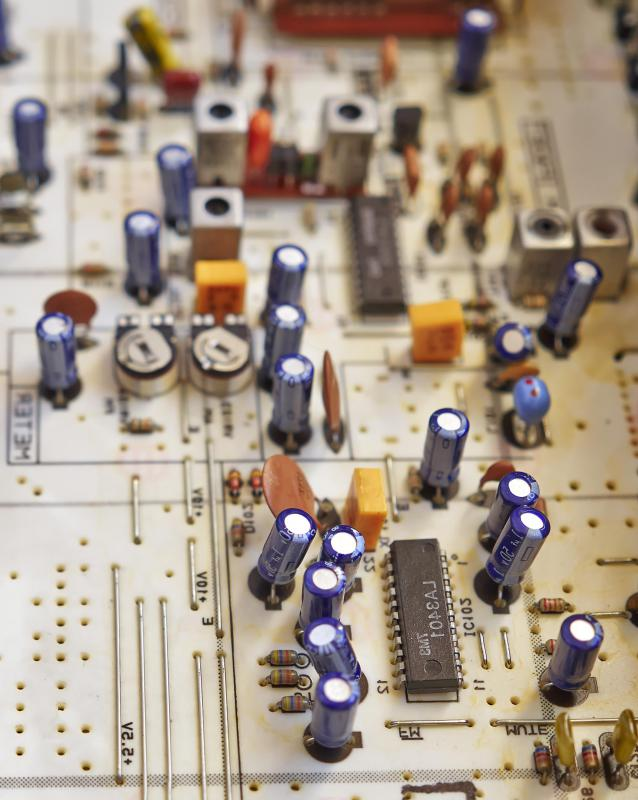# What Is the Purpose of Capacitors in Parallel?

Paul Scott

Groups of capacitors are placed in parallel in a circuit to increase the total capacitance of the group to a value larger than any one of the individual components. Capacitors in parallel exhibit this characteristic due to their tendency to electrically form a single capacitor with a total plate area equal to all of the individual capacitor's plates combined when connected in this configuration. As the capacitance of any capacitor is a product of the component's plate area, this increase causes an overall increase in the combined component's capacitance. When connected in series, the opposite happens, with the total capacitance of the group being smaller than any one of the individual components. This phenomenon is used in applications such as the smoothing of direct-current (DC) output voltages in power supplies.Capacitors in parallel can increase the total capacitance of the group.

Capacitors are electronic components that store electrical energy, usually consisting of thin metal plates separated by a insulating material. The capacitor's ability to store energy is known as capacitance and is expressed in farads (F). Capacitance is largely dictated by the surface area of the capacitor plates and increases with an increase in plate area. This characteristic is harnessed in various ways by either placing groups of capacitors in parallel or series with each other in a circuit. This is also often done with resisters, but with exactly the opposite results in each configuration.A resistor is an electronic component that can lower a circuit’s voltage and its flow of electrical current, and a capacitor is a component that can store electrical charges.

In direct contrast with resistors, capacitors in parallel increase in capacitance where parallel groups of resistors decrease in overall resistance. Conversely, capacitors placed in series see a decrease in capacitance where resistance increases in series resistor arrays. Placing capacitors in parallel is a handy method of increasing the overall capacitance of the group. When placed in parallel, capacitors effectively become one component electrically with a plate area equal to the combined plate areas of all of the components in the group. This means that the total capacitance of a group of capacitors is then larger than any one of its individual members.

The increase in total capacitance of groups of capacitors in parallel is useful in circuits such as DC power supplies. In this application, several capacitors are placed in parallel across the output of a rectified power supply. There, they absorb much of the residual alternating-current (AC) ripple from the output, resulting in a smoother DC power supply. In this way, the circuit designer can maintain all of the other electrical characteristics of the smaller components while increasing the overall capacitance, and, with it, the efficacy of the smoothing capacitors.

## You might also Like